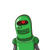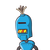# 1. Observe the following pattern(1×2) + (2×3) = (2×3×4)/3(1×2) + (2×3) + (3×4) = (3×4×5)/3(1×2

1. Observe the following pattern

(1×2) + (2×3) = (2×3×4)/3

(1×2) + (2×3) + (3×4) = (3×4×5)/3

(1×2) + (2×3) + (3×4) + (4×5) = (4×5×6)/3

And find the value of

(1×2) + (2×3) + (3×4) + (4×5) + (5×6) Class 8 chapter 6 square and square root​

### 2 thoughts on “1. Observe the following pattern<br /><br />(1×2) + (2×3) = (2×3×4)/3<br /><br />(1×2) + (2×3) + (3×4) = (3×4×5)/3<br /><br />(1×2”

1.2.